# 10.5 Polar form of complex numbers  (Page 4/8)

 Page 4 / 8

## Finding the n Th root of a complex number

Evaluate the cube roots of $\text{\hspace{0.17em}}z=8\left(\mathrm{cos}\left(\frac{2\pi }{3}\right)+i\mathrm{sin}\left(\frac{2\pi }{3}\right)\right).$

We have

$\begin{array}{l}{z}^{\frac{1}{3}}={8}^{\frac{1}{3}}\left[\mathrm{cos}\left(\frac{\frac{2\pi }{3}}{3}+\frac{2k\pi }{3}\right)+i\mathrm{sin}\left(\frac{\frac{2\pi }{3}}{3}+\frac{2k\pi }{3}\right)\right]\hfill \\ {z}^{\frac{1}{3}}=2\left[\mathrm{cos}\left(\frac{2\pi }{9}+\frac{2k\pi }{3}\right)+i\mathrm{sin}\left(\frac{2\pi }{9}+\frac{2k\pi }{3}\right)\right]\hfill \end{array}$

There will be three roots: $\text{\hspace{0.17em}}k=0,\text{\hspace{0.17em}}\text{\hspace{0.17em}}1,\text{\hspace{0.17em}}\text{\hspace{0.17em}}2.\text{\hspace{0.17em}}$ When $\text{\hspace{0.17em}}k=0,\text{\hspace{0.17em}}$ we have

${z}^{\frac{1}{3}}=2\left(\mathrm{cos}\left(\frac{2\pi }{9}\right)+i\mathrm{sin}\left(\frac{2\pi }{9}\right)\right)$

When $\text{\hspace{0.17em}}k=1,\text{\hspace{0.17em}}$ we have

When $\text{\hspace{0.17em}}k=2,\text{\hspace{0.17em}}$ we have

Remember to find the common denominator to simplify fractions in situations like this one. For $\text{\hspace{0.17em}}k=1,\text{\hspace{0.17em}}$ the angle simplification is

$\begin{array}{l}\frac{\frac{2\pi }{3}}{3}+\frac{2\left(1\right)\pi }{3}=\frac{2\pi }{3}\left(\frac{1}{3}\right)+\frac{2\left(1\right)\pi }{3}\left(\frac{3}{3}\right)\hfill \\ \text{\hspace{0.17em}}\text{\hspace{0.17em}}\text{\hspace{0.17em}}\text{\hspace{0.17em}}\text{\hspace{0.17em}}\text{\hspace{0.17em}}\text{\hspace{0.17em}}\text{\hspace{0.17em}}\text{\hspace{0.17em}}\text{\hspace{0.17em}}\text{\hspace{0.17em}}\text{\hspace{0.17em}}\text{\hspace{0.17em}}\text{\hspace{0.17em}}\text{\hspace{0.17em}}\text{\hspace{0.17em}}\text{\hspace{0.17em}}\text{\hspace{0.17em}}\text{\hspace{0.17em}}\text{\hspace{0.17em}}\text{\hspace{0.17em}}\text{\hspace{0.17em}}\text{\hspace{0.17em}}\text{\hspace{0.17em}}\text{\hspace{0.17em}}\text{\hspace{0.17em}}=\frac{2\pi }{9}+\frac{6\pi }{9}\hfill \\ \text{\hspace{0.17em}}\text{\hspace{0.17em}}\text{\hspace{0.17em}}\text{\hspace{0.17em}}\text{\hspace{0.17em}}\text{\hspace{0.17em}}\text{\hspace{0.17em}}\text{\hspace{0.17em}}\text{\hspace{0.17em}}\text{\hspace{0.17em}}\text{\hspace{0.17em}}\text{\hspace{0.17em}}\text{\hspace{0.17em}}\text{\hspace{0.17em}}\text{\hspace{0.17em}}\text{\hspace{0.17em}}\text{\hspace{0.17em}}\text{\hspace{0.17em}}\text{\hspace{0.17em}}\text{\hspace{0.17em}}\text{\hspace{0.17em}}\text{\hspace{0.17em}}\text{\hspace{0.17em}}\text{\hspace{0.17em}}\text{\hspace{0.17em}}\text{\hspace{0.17em}}=\frac{8\pi }{9}\hfill \end{array}$

Find the four fourth roots of $\text{\hspace{0.17em}}16\left(\mathrm{cos}\left(120°\right)+i\mathrm{sin}\left(120°\right)\right).$

${z}_{0}=2\left(\mathrm{cos}\left(30°\right)+i\mathrm{sin}\left(30°\right)\right)$

${z}_{1}=2\left(\mathrm{cos}\left(120°\right)+i\mathrm{sin}\left(120°\right)\right)$

${z}_{2}=2\left(\mathrm{cos}\left(210°\right)+i\mathrm{sin}\left(210°\right)\right)$

${z}_{3}=2\left(\mathrm{cos}\left(300°\right)+i\mathrm{sin}\left(300°\right)\right)$

Access these online resources for additional instruction and practice with polar forms of complex numbers.

## Key concepts

• Complex numbers in the form $\text{\hspace{0.17em}}a+bi\text{\hspace{0.17em}}$ are plotted in the complex plane similar to the way rectangular coordinates are plotted in the rectangular plane. Label the x- axis as the real axis and the y- axis as the imaginary axis. See [link] .
• The absolute value of a complex number is the same as its magnitude. It is the distance from the origin to the point: $\text{\hspace{0.17em}}|z|=\sqrt{{a}^{2}+{b}^{2}}.\text{\hspace{0.17em}}$ See [link] and [link] .
• To write complex numbers in polar form, we use the formulas $\text{\hspace{0.17em}}x=r\mathrm{cos}\text{\hspace{0.17em}}\theta ,y=r\mathrm{sin}\text{\hspace{0.17em}}\theta ,\text{\hspace{0.17em}}$ and $\text{\hspace{0.17em}}r=\sqrt{{x}^{2}+{y}^{2}}.\text{\hspace{0.17em}}$ Then, $\text{\hspace{0.17em}}z=r\left(\mathrm{cos}\text{\hspace{0.17em}}\theta +i\mathrm{sin}\text{\hspace{0.17em}}\theta \right).\text{\hspace{0.17em}}$ See [link] and [link] .
• To convert from polar form to rectangular form, first evaluate the trigonometric functions. Then, multiply through by $\text{\hspace{0.17em}}r.\text{\hspace{0.17em}}$ See [link] and [link] .
• To find the product of two complex numbers, multiply the two moduli and add the two angles. Evaluate the trigonometric functions, and multiply using the distributive property. See [link] .
• To find the quotient of two complex numbers in polar form, find the quotient of the two moduli and the difference of the two angles. See [link] .
• To find the power of a complex number $\text{\hspace{0.17em}}{z}^{n},\text{\hspace{0.17em}}$ raise $\text{\hspace{0.17em}}r\text{\hspace{0.17em}}$ to the power $\text{\hspace{0.17em}}n,$ and multiply $\text{\hspace{0.17em}}\theta \text{\hspace{0.17em}}$ by $\text{\hspace{0.17em}}n.\text{\hspace{0.17em}}$ See [link] .
• Finding the roots of a complex number is the same as raising a complex number to a power, but using a rational exponent. See [link] .

## Verbal

A complex number is $\text{\hspace{0.17em}}a+bi.\text{\hspace{0.17em}}$ Explain each part.

a is the real part, b is the imaginary part, and $\text{\hspace{0.17em}}i=\sqrt{-1}$

What does the absolute value of a complex number represent?

How is a complex number converted to polar form?

Polar form converts the real and imaginary part of the complex number in polar form using $\text{\hspace{0.17em}}x=r\mathrm{cos}\theta \text{\hspace{0.17em}}$ and $\text{\hspace{0.17em}}y=r\mathrm{sin}\theta .$

How do we find the product of two complex numbers?

What is De Moivre’s Theorem and what is it used for?

${z}^{n}={r}^{n}\left(\mathrm{cos}\left(n\theta \right)+i\mathrm{sin}\left(n\theta \right)\right)\text{\hspace{0.17em}}$ It is used to simplify polar form when a number has been raised to a power.

## Algebraic

For the following exercises, find the absolute value of the given complex number.

$5+\text{​}3i$

$-7+\text{​}i$

$5\sqrt{2}$

$-3-3i$

$\sqrt{2}-6i$

$\sqrt{38}$

$2i$

$2.2-3.1i$

$\sqrt{14.45}$

For the following exercises, write the complex number in polar form.

$2+2i$

$8-4i$

$4\sqrt{5}\mathrm{cis}\left(333.4°\right)$

$-\frac{1}{2}-\frac{1}{2}\text{​}i$

$\sqrt{3}+i$

$2\mathrm{cis}\left(\frac{\pi }{6}\right)$

$3i$

For the following exercises, convert the complex number from polar to rectangular form.

$z=7\mathrm{cis}\left(\frac{\pi }{6}\right)$

$\frac{7\sqrt{3}}{2}+i\frac{7}{2}$

$z=2\mathrm{cis}\left(\frac{\pi }{3}\right)$

$z=4\mathrm{cis}\left(\frac{7\pi }{6}\right)$

$-2\sqrt{3}-2i$

$z=7\mathrm{cis}\left(25°\right)$

$z=3\mathrm{cis}\left(240°\right)$

$-1.5-i\frac{3\sqrt{3}}{2}$

$z=\sqrt{2}\mathrm{cis}\left(100°\right)$

For the following exercises, find $\text{\hspace{0.17em}}{z}_{1}{z}_{2}\text{\hspace{0.17em}}$ in polar form.

$4\sqrt{3}\mathrm{cis}\left(198°\right)$

$\frac{3}{4}\mathrm{cis}\left(180°\right)$

$5\sqrt{3}\mathrm{cis}\left(\frac{17\pi }{24}\right)$

For the following exercises, find $\text{\hspace{0.17em}}\frac{{z}_{1}}{{z}_{2}}\text{\hspace{0.17em}}$ in polar form.

$7\mathrm{cis}\left(70°\right)$

$5\mathrm{cis}\left(80°\right)$

$5\mathrm{cis}\left(\frac{\pi }{3}\right)$

For the following exercises, find the powers of each complex number in polar form.

Find $\text{\hspace{0.17em}}{z}^{3}\text{\hspace{0.17em}}$ when $\text{\hspace{0.17em}}z=5\mathrm{cis}\left(45°\right).$

$125\mathrm{cis}\left(135°\right)$

Find $\text{\hspace{0.17em}}{z}^{4}\text{\hspace{0.17em}}$ when $\text{\hspace{0.17em}}z=2\mathrm{cis}\left(70°\right).$

Find $\text{\hspace{0.17em}}{z}^{2}\text{\hspace{0.17em}}$ when $\text{\hspace{0.17em}}z=3\mathrm{cis}\left(120°\right).$

$9\mathrm{cis}\left(240°\right)$

Find $\text{\hspace{0.17em}}{z}^{2}\text{\hspace{0.17em}}$ when $\text{\hspace{0.17em}}z=4\mathrm{cis}\left(\frac{\pi }{4}\right).$

Find $\text{\hspace{0.17em}}{z}^{4}\text{\hspace{0.17em}}$ when $\text{\hspace{0.17em}}z=\mathrm{cis}\left(\frac{3\pi }{16}\right).$

$\mathrm{cis}\left(\frac{3\pi }{4}\right)$

Find $\text{\hspace{0.17em}}{z}^{3}\text{\hspace{0.17em}}$ when $\text{\hspace{0.17em}}z=3\mathrm{cis}\left(\frac{5\pi }{3}\right).$

For the following exercises, evaluate each root.

Evaluate the cube root of $\text{\hspace{0.17em}}z\text{\hspace{0.17em}}$ when $\text{\hspace{0.17em}}z=27\mathrm{cis}\left(240°\right).$

$\text{\hspace{0.17em}}3\mathrm{cis}\left(80°\right),3\mathrm{cis}\left(200°\right),3\mathrm{cis}\left(320°\right)\text{\hspace{0.17em}}$

Evaluate the square root of $\text{\hspace{0.17em}}z\text{\hspace{0.17em}}$ when $\text{\hspace{0.17em}}z=16\mathrm{cis}\left(100°\right).$

Evaluate the cube root of $\text{\hspace{0.17em}}z\text{\hspace{0.17em}}$ when $\text{\hspace{0.17em}}z=32\mathrm{cis}\left(\frac{2\pi }{3}\right).$

$\text{\hspace{0.17em}}2\sqrt{4}\mathrm{cis}\left(\frac{2\pi }{9}\right),2\sqrt{4}\mathrm{cis}\left(\frac{8\pi }{9}\right),2\sqrt{4}\mathrm{cis}\left(\frac{14\pi }{9}\right)$

Evaluate the square root of $\text{\hspace{0.17em}}z\text{\hspace{0.17em}}$ when $\text{\hspace{0.17em}}z=32\text{cis}\left(\pi \right).$

Evaluate the cube root of $\text{\hspace{0.17em}}z\text{\hspace{0.17em}}$ when $\text{\hspace{0.17em}}z=8\mathrm{cis}\left(\frac{7\pi }{4}\right).$

$2\sqrt{2}\mathrm{cis}\left(\frac{7\pi }{8}\right),2\sqrt{2}\mathrm{cis}\left(\frac{15\pi }{8}\right)$

## Graphical

For the following exercises, plot the complex number in the complex plane.

$2+4i$

$-3-3i$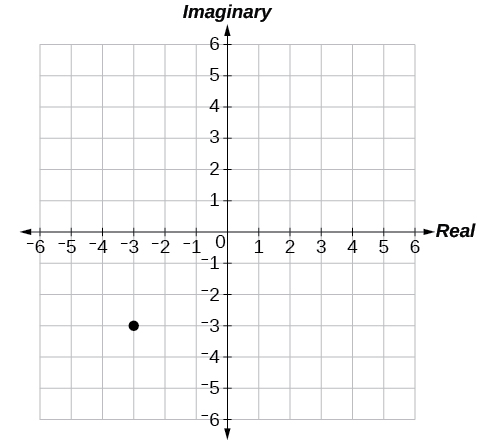$5-4i$

$-1-5i$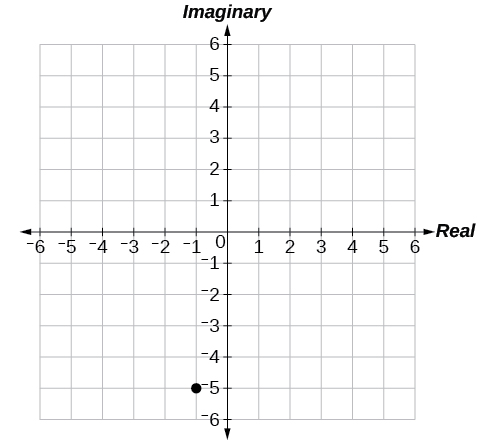$3+2i$

$2i$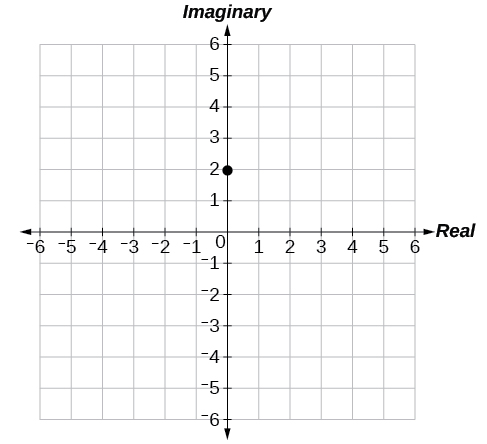$-4$

$6-2i$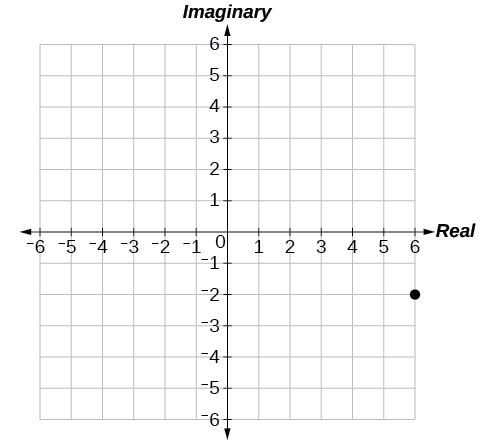$-2+i$

$1-4i$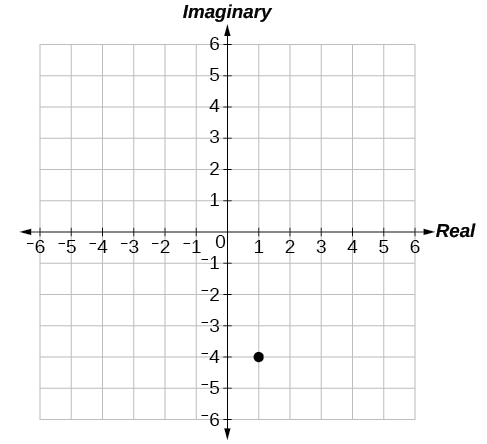## Technology

For the following exercises, find all answers rounded to the nearest hundredth.

Use the rectangular to polar feature on the graphing calculator to change $\text{\hspace{0.17em}}5+5i\text{\hspace{0.17em}}$ to polar form.

Use the rectangular to polar feature on the graphing calculator to change $\text{\hspace{0.17em}}3-2i\text{\hspace{0.17em}}$ to polar form.

$\text{\hspace{0.17em}}3.61{e}^{-0.59i}\text{\hspace{0.17em}}$

Use the rectangular to polar feature on the graphing calculator to change $-3-8i\text{\hspace{0.17em}}$ to polar form.

Use the polar to rectangular feature on the graphing calculator to change $\text{\hspace{0.17em}}4\mathrm{cis}\left(120°\right)\text{\hspace{0.17em}}$ to rectangular form.

$\text{\hspace{0.17em}}-2+3.46i\text{\hspace{0.17em}}$

Use the polar to rectangular feature on the graphing calculator to change $\text{\hspace{0.17em}}2\mathrm{cis}\left(45°\right)\text{\hspace{0.17em}}$ to rectangular form.

Use the polar to rectangular feature on the graphing calculator to change $\text{\hspace{0.17em}}5\mathrm{cis}\left(210°\right)\text{\hspace{0.17em}}$ to rectangular form.

$\text{\hspace{0.17em}}-4.33-2.50i\text{\hspace{0.17em}}$

root under 3-root under 2 by 5 y square
The sum of the first n terms of a certain series is 2^n-1, Show that , this series is Geometric and Find the formula of the n^th
cosA\1+sinA=secA-tanA
why two x + seven is equal to nineteen.
The numbers cannot be combined with the x
Othman
2x + 7 =19
humberto
2x +7=19. 2x=19 - 7 2x=12 x=6
Yvonne
because x is 6
SAIDI
what is the best practice that will address the issue on this topic? anyone who can help me. i'm working on my action research.
simplify each radical by removing as many factors as possible (a) √75
how is infinity bidder from undefined?
what is the value of x in 4x-2+3
give the complete question
Shanky
4x=3-2 4x=1 x=1+4 x=5 5x
Olaiya
hi can you give another equation I'd like to solve it
Daniel
what is the value of x in 4x-2+3
Olaiya
if 4x-2+3 = 0 then 4x = 2-3 4x = -1 x = -(1÷4) is the answer.
Jacob
4x-2+3 4x=-3+2 4×=-1 4×/4=-1/4
LUTHO
then x=-1/4
LUTHO
4x-2+3 4x=-3+2 4x=-1 4x÷4=-1÷4 x=-1÷4
LUTHO
A research student is working with a culture of bacteria that doubles in size every twenty minutes. The initial population count was  1350  bacteria. Rounding to five significant digits, write an exponential equation representing this situation. To the nearest whole number, what is the population size after  3  hours?
v=lbh calculate the volume if i.l=5cm, b=2cm ,h=3cm
Need help with math
Peya
can you help me on this topic of Geometry if l help you
litshani
( cosec Q _ cot Q ) whole spuare = 1_cosQ / 1+cosQ
A guy wire for a suspension bridge runs from the ground diagonally to the top of the closest pylon to make a triangle. We can use the Pythagorean Theorem to find the length of guy wire needed. The square of the distance between the wire on the ground and the pylon on the ground is 90,000 feet. The square of the height of the pylon is 160,000 feet. So, the length of the guy wire can be found by evaluating √(90000+160000). What is the length of the guy wire?
the indicated sum of a sequence is known as
how do I attempted a trig number as a starter
cos 18 ____ sin 72 evaluateByByBy Janet ForresterBy Olivia D'AmbrogioBy Brooke DelaneyBy OpenStaxBy OpenStaxBy Stephen VoronBy Brooke DelaneyBy Sandhills MLTBy Yacoub JayoghliBy OpenStax